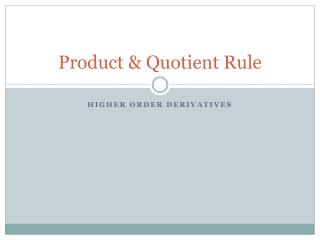# Product & Quotient Rule - PowerPoint PPT PresentationDownload PresentationProduct & Quotient Rule

Product & Quotient Rule
Download Presentation## Product & Quotient Rule

- - - - - - - - - - - - - - - - - - - - - - - - - - - E N D - - - - - - - - - - - - - - - - - - - - - - - - - - -
##### Presentation Transcript

1. Product & Quotient Rule Higher Order Derivatives

2. The Product Rule • Theorem. Let f and g be differentiable functions. Then the derivative of the product fg is • (fg) '(x) = f(x) g '(x) + g(x) f '(x) In other words, first times the derivative of the second plus second times the derivative of the first.

3. Using the Product Rule • Example:

4. Product Rule • Example:

5. Product Rule • Example

6. Quotient Rule • Theorem. Let f and g be differentiable functions. Then the derivative of the quotient f/g is In other words, low d high minus high d low over low low.

7. Quotient Rule • Example • Find the derivative of

8. Rewriting Before Differentiating • Example:

9. Derivatives of Trigonometric Functions

10. Proof of Derivative of Tangent • Considering

11. Differentiating Trig Functions • y = x – tan x • y = x sec x y’ = x(sec x tan x) + sec x (1) = sec x (x tan x + 1)

12. Differentiating Trigonometric Functions

13. Higher Order Derivatives Applications: Finding Acceleration Due to Gravity Population Growth p. 125 problem 79 Any time we are asked to find the rate at which a rate is changing, this is a second derivative.